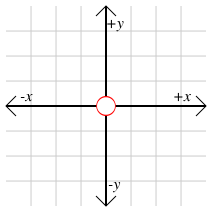xOrigin (mathematics)EncyclopediaIn mathematics
Mathematics
Mathematics is the study of quantity, space, structure, and change. Mathematicians seek out patterns and formulate new conjectures. Mathematicians resolve the truth or falsity of conjectures by mathematical proofs, which are arguments sufficient to convince other mathematicians of their validity...

, the origin of a Euclidean space
Euclidean space
In mathematics, Euclidean space is the Euclidean plane and three-dimensional space of Euclidean geometry, as well as the generalizations of these notions to higher dimensions...

is a special point
Point (geometry)
In geometry, topology and related branches of mathematics a spatial point is a primitive notion upon which other concepts may be defined. In geometry, points are zero-dimensional; i.e., they do not have volume, area, length, or any other higher-dimensional analogue. In branches of mathematics...

, usually denoted by the letter O, used as a fixed point of reference for the geometry of the surrounding space. In a Cartesian coordinate system
Cartesian coordinate system
A Cartesian coordinate system specifies each point uniquely in a plane by a pair of numerical coordinates, which are the signed distances from the point to two fixed perpendicular directed lines, measured in the same unit of length...

, the origin is the point where the axes of the system intersect. In Euclidean geometry
Euclidean geometry
Euclidean geometry is a mathematical system attributed to the Alexandrian Greek mathematician Euclid, which he described in his textbook on geometry: the Elements. Euclid's method consists in assuming a small set of intuitively appealing axioms, and deducing many other propositions from these...

, the origin may be chosen freely as any convenient point of reference.

The most common coordinate systems are two-dimensional (contained in a plane
Plane (mathematics)
In mathematics, a plane is a flat, two-dimensional surface. A plane is the two dimensional analogue of a point , a line and a space...

) and three-dimensional (contained in a space
Space
Space is the boundless, three-dimensional extent in which objects and events occur and have relative position and direction. Physical space is often conceived in three linear dimensions, although modern physicists usually consider it, with time, to be part of a boundless four-dimensional continuum...

), composed of two and three perpendicular
Perpendicular
In geometry, two lines or planes are considered perpendicular to each other if they form congruent adjacent angles . The term may be used as a noun or adjective...

axes, respectively. The origin divides each of these axes into two halves, a positive and a negative semiaxis. Points can then be located with reference to the origin by giving their numerical coordinates—that is, the positions of their projections along each axis, either in the positive or negative direction. The coordinates of the origin are always all zero, for example (0,0) in two dimensions and (0,0,0) in three.

The origin of the complex plane
Complex plane
In mathematics, the complex plane or z-plane is a geometric representation of the complex numbers established by the real axis and the orthogonal imaginary axis...

can be referred as the point where real axis and imaginary axis intersect each other. In other words, it is the point representing 0 + 0i.

## Symmetry with respect to the originWhen a graph is symmetric with respect to the origin, it describes a graph that looks the same before and after the graph is rotated 180 degrees. Formally, a graph is symmetric with respect to the origin if it is unchanged when reflected across both the x-axis and y-axis.

The graphs of an invertible function and its inverse always have the line y = x which definitely passes through the origin as the axis of symmetry.